Subtraction of integers using number line

Chapter 6 Class 6 Integers
Concept wise

#### Subtraction 3 & 5 on number line

3 − 5 = 3 + (−5)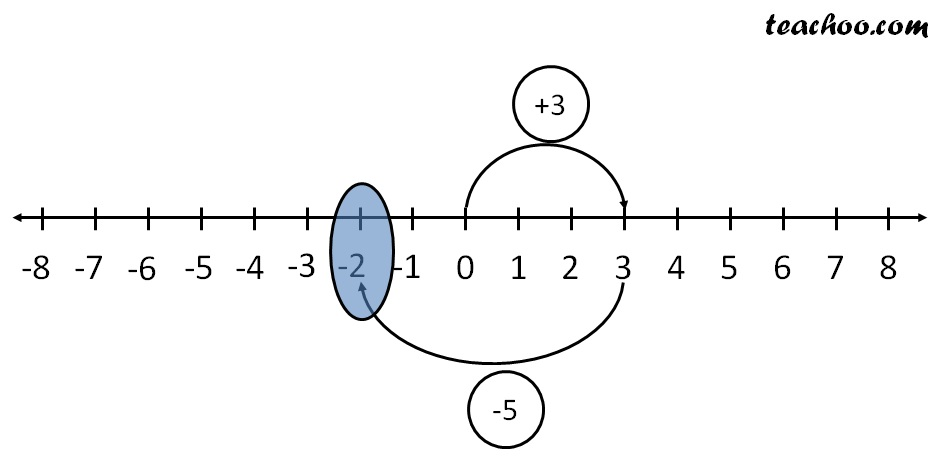First we move 3 steps to the right from 0

And then , we move 5 steps to the left

we reach −2

∴    3  −  5 = −2

#### Subtract (−3) & (−5) on number line

(−3) − (−5) = (−3) + 5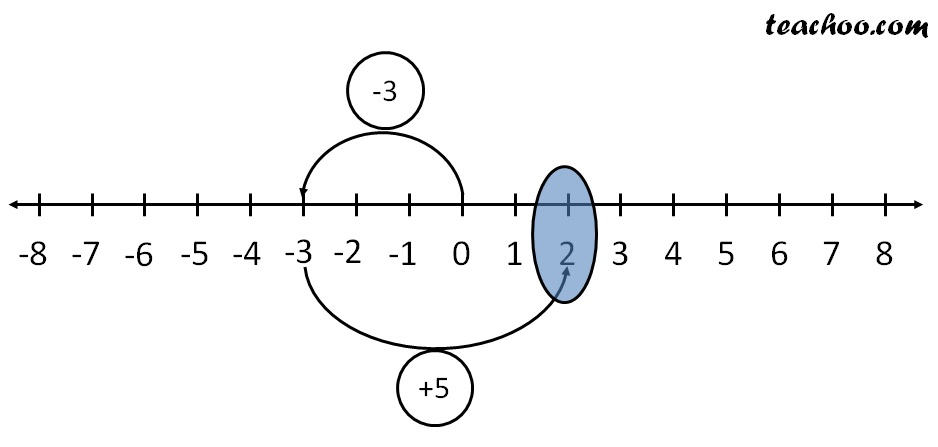First we move 3 steps to the left from 0

And then , we move 5 steps to the right

we reach 2

∴ (−3) − (−5) = 2

#### Subtract (−3) & (5) on number line

(−3) − 5 = (−3) + (−5)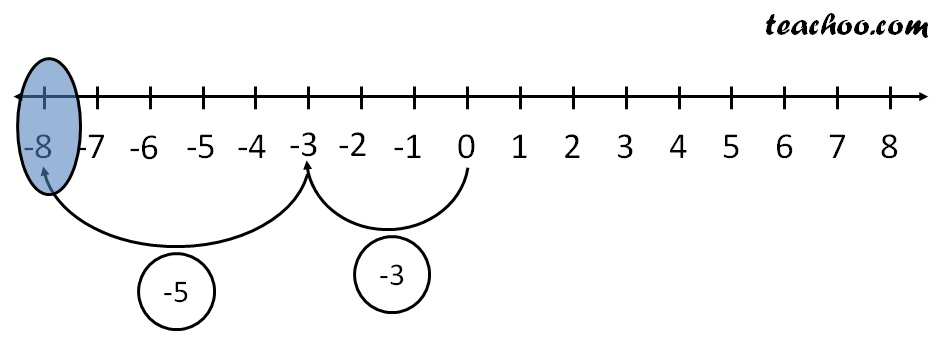First we move 3 steps to the left from 0

And then , we move 5 steps to the left

we reach − 8

∴   (− 3) − 5 = −8

Subtract 3 & (−5) on number line

3 − (−5) = 3 + 5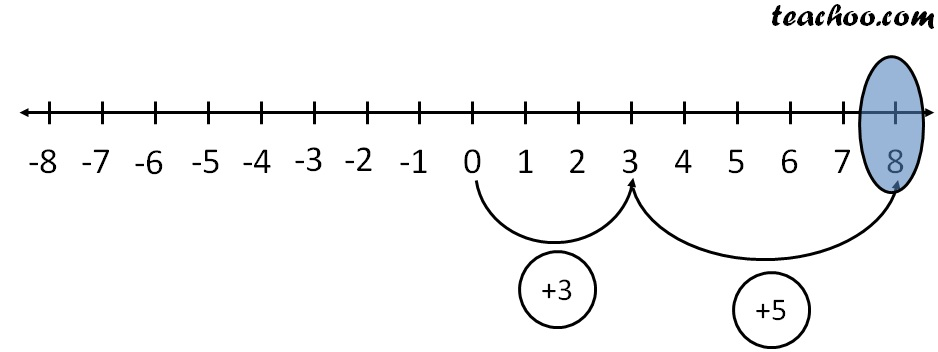First we move 3 steps to the right from 0

And then , we move 5 steps to the right

we reach 8

∴ 3 − (−5) = 8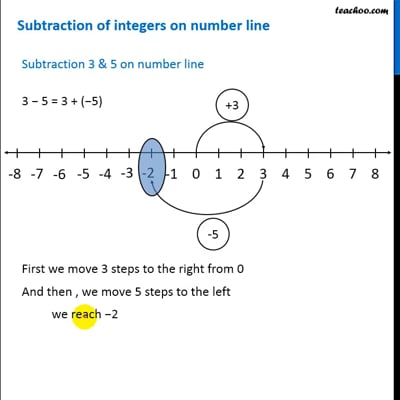This video is only available for Teachoo black users